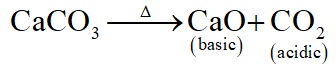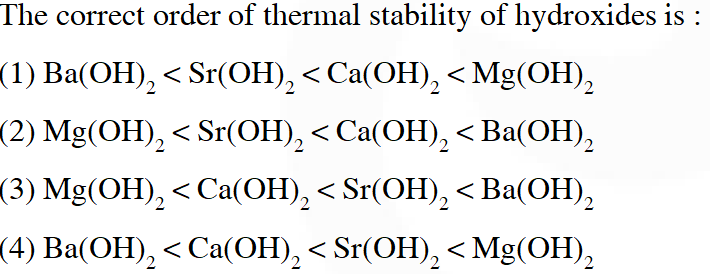# s-block - JEE Main Previous Year Questions with Solutions

JEE Main Previous Year Papers Questions of Chemistry With Solutions are available at eSaral. Simulator   Previous Years AIEEE/JEE Mains Questions
Q. The products obtained on heating $\mathrm{LiNO}_{3}$ will be :- (1) $\mathrm{LiNO}_{2}+\mathrm{O}_{2}$ (2) $\mathrm{Li}_{2} \mathrm{O}+\mathrm{NO}_{2}+\mathrm{O}_{2}$ (3) $\mathrm{Li}_{3} \mathrm{N}+\mathrm{O}_{2}$ (4) $\mathrm{Li}_{2} \mathrm{O}+\mathrm{NO}+\mathrm{O}_{2}$ [AIEEE-2011]
Ans. (2) Fact based.
Q. What is the best description of the change that occurs when $\mathrm{Na}_{2} \mathrm{O}(\mathrm{s})$ is dissolved in water ? (1) Oxidation number of sodium decreases (2) Oxide ion accepts sharing in a pair of electrons (3) Oxide ion donates a pair of electrons (4) Oxidation number of oxygen increases
Ans. (3) $\mathrm{O}^{-2}+\mathrm{H}^{\ominus} \rightarrow \mathrm{O}-\mathrm{H}\left\{\mathrm{O}^{-2} \rightarrow \mathrm{H}^{\ominus}\right\}$
Q. Which of the following on thermal-decomposition yields a basic as well as an acidic oxide ? (1) $\mathrm{NH}_{4} \mathrm{NO}_{3}$ (2) NaNO $_{3}$ (3) $\mathrm{KClO}_{3}$ (4) CaCO $_{3}$ [AIEEE-2012]
Ans. (4)Q. Fire extinguishers contain $\mathrm{H}_{2} \mathrm{SO}_{4}$ and which one of the following :- (1) $\mathrm{CaCO}_{3}$ (2) $\mathrm{NaHCO}_{3}$ and $\mathrm{Na}_{2} \mathrm{CO}_{3}$ (3) $\mathrm{Na}_{2} \mathrm{CO}_{3}$ (4) $\mathrm{NaHCO}_{3}$ [JEE MAIN-2012, Online]
Ans. (4) Solution not required
Q. Based on lattice energy and other considerations, which one of the following alkali metal chloride is expected to have the highest melting point ? (1) RbCl (2) LiCl (3) KCl (4) NaCl [JEE MAIN-2012, Online]
Ans. (4) NaCl having highest lattice energy have highest melting point among these.
Q. Which one of the following will react most vigorously with water ? (1) Li (2) K (3) Rb (4) Na [JEE MAIN-2012, Online]
Ans. (3) Down the group reactivity of s-block metals increases.
Q. A metal M on heating in nitrogen gas gives Y. Y on treatment with $\mathrm{H}_{2} \mathrm{O}$ gives a colourless gas which when passed through $\mathrm{CuSO}_{4}$ solution gives a blue colour, Y is :- (1) $\mathrm{NH}_{3}$ (2) MgO (3) $\mathrm{Mg}_{3} \mathrm{N}_{2}$ (4) $\mathrm{Mg}\left(\mathrm{NO}_{3}\right)_{2}$ [JEE MAIN-2012, Online]
Ans. (3) $\mathrm{M}+\mathrm{N}_{2} \rightarrow$ metal nitride (y) so according to the given options answer is (3)
Q. The correct statement for the molecule, $\mathrm{CSI}_{3}$, is : (1) it contains $\mathrm{Cs}^{3+}$ and I– ions (2) it contains $\mathrm{Cs}^{+}$, I– and lattice I2 molecule (3) it is a covalent molecule (4) it contains $\mathrm{Cs}^{+}$ and ions. [JEE(Main)-2014]
Ans. (4) $\mathrm{CsI}_{3}$ is ionic compound which have $\mathrm{Cs}^{\oplus}$ and $\mathrm{I}_{3}^{\oplus}$ ion.
Q. Which of the following statements about $\mathrm{Na}_{2} \mathrm{O}_{2}$ is not correct ? (1) $\mathrm{Na}_{2} \mathrm{O}_{2}$ oxidises $\mathrm{Cr}^{3+}$ to $\mathrm{CrO}_{4}^{2-}$ in acid medium (2) It is diamagnetic in nature (3) It is the super oxide of sodium (4) It is a derivative of $\mathrm{H}_{2} \mathrm{O}_{2}$ [JEE MAIN-2014, Online]
Ans. (3) $\mathrm{Na}_{2} \mathrm{O}_{2}$ is sodium peroxide not the superoxide.
Q. Amongst LiCl, RbCl, $\mathrm{BeCl}_{2}$ and $\mathrm{MgCl}_{2}$ the compounds with the greatest and the least ionic character, respectively are : (1) $\mathrm{RbCl}$ and $\mathrm{MgCl}_{2}$ (2) LiCl and RbCl (3) $\mathrm{MgCl}_{2}$ and $\mathrm{BeCl}_{2}$ (4) $\mathrm{RbCl}$ and $\mathrm{BeCl}_{2}$ [JEE MAIN-2014, Online]
Ans. (4) Due to smallest cationic size $\mathrm{BeCl}_{2}$ is least ionic while due to biggest ionic size of $\mathrm{Rb}^{\oplus}$, RbCl has greatest ionic character.
Q. The correct order of thermal stability of hydroxides is :[JEE(Main)Online-2015]
Ans. (3) For the ionic compound having polyatomic anion, thermal stability increases down the group.
Q. Which of the alkaline earth metal halide given below is essentially covalent in nature :- (1) $\mathrm{SrCl}_{2}$ (2) $\mathrm{CaCl}_{2}$ (3) $\mathrm{BeCl}_{2}$ (4) $\mathrm{MgCl}_{2}$ [JEE(Main)Online-2015]
Ans. (3) Fact based.

Purna chandar
July 12, 2022, 4 p.m.
Vere level super sir 🤩🤩
July 12, 2022, 3:59 p.m.
Vere level super sir 🤩🤩
Dev
June 23, 2021, 5:18 p.m.
need more questions
Aryan
May 27, 2021, 3:30 p.m.
Vidya
Feb. 11, 2021, 7:18 a.m.
If you please provide more questions it would be more helpful to us.....
Sandhya Ramesh
Dec. 20, 2020, 12:42 a.m.
mohan
Nov. 27, 2020, 7:19 a.m.
pls provide more questions.thankyou
mohan
Nov. 27, 2020, 7:18 a.m.
pls provide more questions
Anupam Palui
Oct. 27, 2020, 8:34 p.m.
Anupam Palui
Oct. 27, 2020, 8:05 p.m.
Devika
Oct. 24, 2020, 10:58 a.m.
Good
Ankana Dutta
Oct. 7, 2020, 1:28 p.m.
Shanmukh
Sept. 30, 2020, 10:05 a.m.
Thank you
NITIN Rao
Sept. 25, 2020, 3 p.m.
himanshu gahlot
Sept. 25, 2020, 10:56 a.m.
please provide recent years questions. thank u
Rohita
Sept. 14, 2020, 2:37 p.m.
good
jack
Sept. 1, 2020, 7:34 p.m.
thankyou!!!!!
jack
Sept. 1, 2020, 7:33 p.m.
lrnoqwnrglqwnroglwnjn
Amisthina
Sept. 1, 2020, 1:13 p.m.
Pls give us a latest questions
Bhanu
Aug. 31, 2020, 9:15 a.m.
Thank you
Abhiram
Aug. 27, 2020, 11:31 a.m.
Awesome questions to boost confidence
kfkorefmeokfke
Aug. 27, 2020, 7:11 a.m.
rr4rrg fkdfkf gfrelkf fmkfee f ekfq kfqfm rmfr rkrkk4 jkfmkefq frfrmkfrr f rk rqtfr girk kmirmtr frfqrkf fkermfkqf qfmmkqr fmoqm3f 4rtmkt4 rt 4tm4t 4t4o kqmrtot4 rt4kmroo4ro4r4 r4krk4rk4r4 4kmro4o4 rm4ro4o4 4ro4or4oo4 ro4o prl43rp4lr roikgiigkjb bifrmfgbkif mi
Ansh
Aug. 24, 2020, 4:26 a.m.
Akanksha
Aug. 23, 2020, 9:18 a.m.
Good questions to practice
Akhila
Aug. 21, 2020, 9:14 a.m.
suhail
Aug. 21, 2020, 7:24 a.m.
thanks for giving these IMPORTANT questions.....
pallavi
Aug. 16, 2020, 9:01 p.m.
Thanks for the questions ... If possible please provide more questions..
Riya
Aug. 5, 2020, 7:32 a.m.
More questions reqired
sanika k
Aug. 1, 2020, 10:08 p.m.
Want more anyways thanks
Rohit lamba
July 24, 2020, 12:26 a.m.
Thanks for providing
Husain
July 13, 2020, 2:05 p.m.
Thanks for providing
roja
July 7, 2020, 10:52 a.m.
super still want more
Rohan
June 22, 2020, 1:15 a.m.
If every year questions were available then it would be more helpful!!!anyways thank you☺☺
mariya
June 14, 2020, 1:03 p.m.
thanqu so much for these valuable question...it is very helpfull for medical aspirants
Yashasvi Sharma
June 9, 2020, 7:52 p.m.
Bhai 2020 tk ke questions post kr dete please
None
Free Study Material